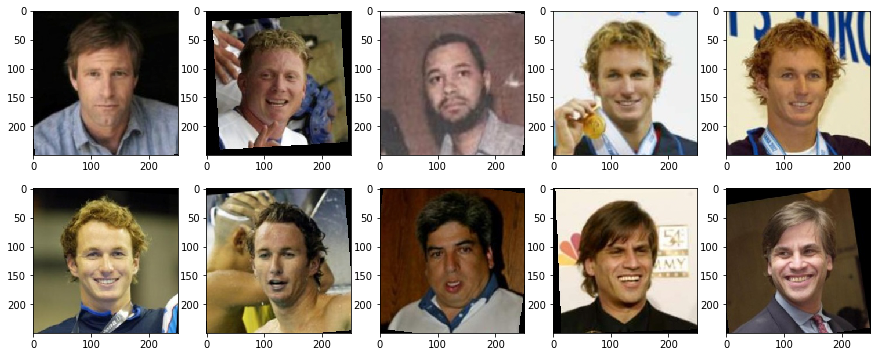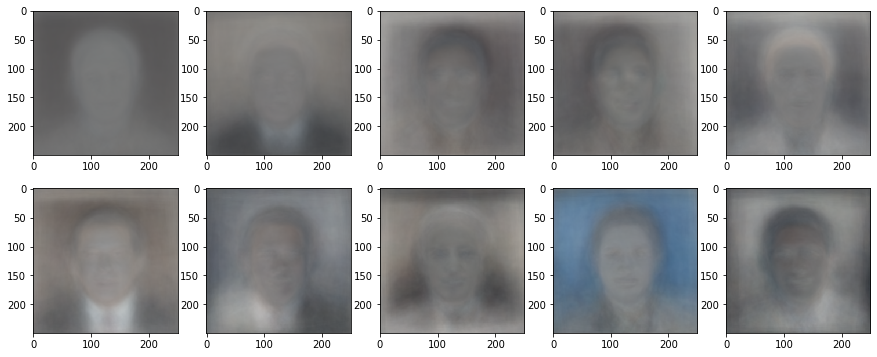# Eigenfaces¶

In this notebook, we apply the deterministic and randomized variants of the SVD to a large, real-life dataset. We will be using the Labeled Faces in the Wild (LFW) dataset, which consists of ~$8000$ RGB images of dimensions $250\times 250$. It's computationally infeasible for us to use the whole dataset, so we load a subset (1000 images).

#### Import packages¶

In :
import numpy as np
from matplotlib import pyplot as plt
from PIL import Image
import glob
from time import perf_counter
from tqdm import tqdm, trange

%matplotlib inline

In :
%cd ..
from rputil import *
%cd -

C:\Users\Sean\random-projections
C:\Users\Sean\random-projections\notebooks


We flatten each image to represent it as vector of length $250\cdot 250 \cdot 3 = 187500$. This will yield a data matrix $A$ of size $187500 \times 1000$.

In :
m = 187500
n = 1000

data = np.empty((m, n))

for i,filename in enumerate(glob.iglob('../datasets/lfw/**/*.jpg', recursive = True)):
if i >= n:
break
im=Image.open(filename)
data[:,i] = np.asarray(im).flatten()
im.close()

In :
data /= 255 # convert from int to float representation


#### Column-center the data¶

This allows us to interpret the data in terms of the way it deviates from an 'average' face.

In :
data_mean = np.expand_dims(np.mean(data, axis=1), 1)
data -= data_mean


#### Calculate eigenfaces¶

We compute the singular value decomposition, $$A = U \Sigma V^* \,,$$ and then truncate $U$, keeping only the first $k$ columns, to use it as a basis for a rank-$k$ approximation.

Similarly, we use random projection to compute a randomized approximation of the SVD.

In :
start = perf_counter()
det_U, det_Sigma, det_Vh = np.linalg.svd(data, full_matrices=False)
end = perf_counter()
det_time = end - start

In :
k = 350

In :
det_basis = det_U[:,:k]
print(det_basis.shape)

(187500, 350)

In :
rand_basis = random_svd_rank_k(data, k, power=0, only_basis=True)
print(rand_basis.shape)

(187500, 350)

In :
def normalize_basis(basis):
return (basis - np.min(basis))/(np.max(basis)-np.min(basis))

In :
normalized_det_basis = normalize_basis(det_basis)

In :
normalized_rand_basis = normalize_basis(rand_basis)


Let's take a look at some of the first few faces in the dataset.

Some things to note:

• there can be multiple pictures of the same person
• the faces are all mostly centered and facing straight, but there are some exceptions
In :
def plot_examples(rows, cols):
fig, axes = plt.subplots(rows, cols, figsize=(15, 15 / cols * rows))
for i,ax in enumerate(axes.flat):
ax.imshow((data[:,i]+np.squeeze(data_mean)).reshape(250,250,3))

In :
plot_examples(2, 5)And now the eigenvectors, or 'eigenfaces,' comprising a basis for the image space:

In :
def plot_eigenfaces(basis, rows, cols):
fig, ax = plt.subplots(rows, cols, figsize=(15, 15 / cols * rows))
for i in range(rows):
for j in range(cols):
ax[i][j].imshow(basis[:,i * cols + j].reshape(250,250,3))

In :
plot_eigenfaces(normalized_det_basis, 2, 5)In :
plot_eigenfaces(normalized_rand_basis, 2, 5)